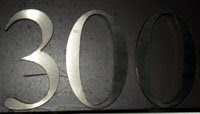## Wednesday, December 10, 2008

### 300

300 = 22 x 3 x 52.

300 is a triangular number, the sum of all positive integers up to 24: 1 + 2 + 3 + . . . + 22 + 23 + 24 = 300.

300 is the sum of twin primes: 149 + 151 = 300.

300 is a number n such that n2 (90000) contains exactly two different digits.In bowling,
300 is the largest possible score.

Source:
Wolfram MathWorld

#### 1 comment:Anonymous said...

300 is sum twin prime wow !